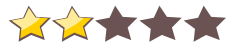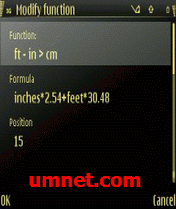Name - My Calcul
Category - systemtools
Resolution - multi
Type - Symbian s60v3
Size - 137 KB
Rating - 2/5 from 18 ratings
Description:
Ver v1.0, for S60v3 SymbianOS9.1 incl Keygen-HSpda

My Calcul is your calculator and nobody else’s: like most other calculator applications, it has a number of function buttons, which perform specific calculations on the number entered or calculated. But unlike in other applications, the buttons in My Calcul can be programmed by you to do exactly the calculations you want. You are free to set the name of each function button and the calculation formula behind it.

The calculation formula behind each button can take several named variables and an unlimited number of parenthesis. In the case of function ft-in>cm, the formula has 2 variables named inches and feet :

Main features of My Calcul :
the calculation formulae can have up to 255 characters, with an unlimited number of parenthesis and named variables. The last value of each variable is memorized within one session, and it is offered as default value when the same variable is used again, in the same formula or in another formula.
the following functions are recognized in formulae: log, ln, factorial, sin, cos, tan, acos, asin, atan, cosh, sinh, acosh, asinh. The numbers pi and e are also recognized.
the calculator can operate in standard algebraic mode or in RPN mode (Reverse Polish Notation).
My Calcul features an equation solver module. When the user loads an equation with n variables (up to 6), the application automatically builds up a table with one line per variable. The table can be used like a small spreadsheet where the user enters values for any sub-set of n-1 variables and let the application calculate the value of the nth variable to solve the equation. Each cell in this special kind of spreadsheet is both an input cell and a calculated cell. This is very useful for all sorts of “what if” type calculations.